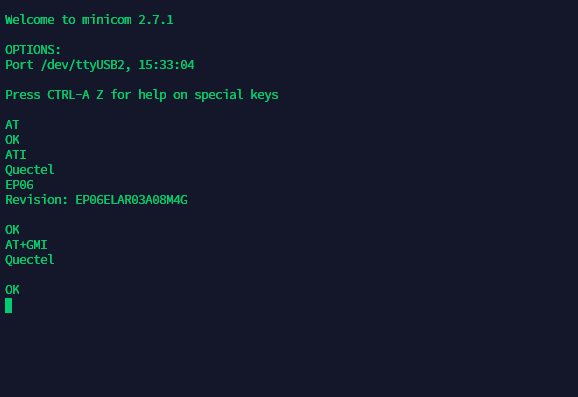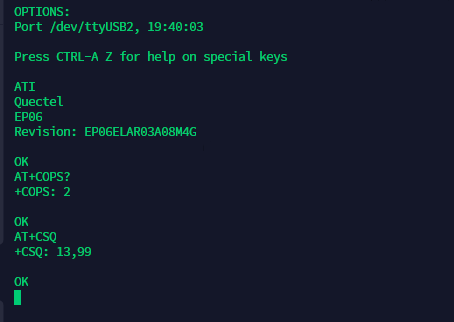# Help to install modem EP06-E with Linux OpenWRT or Windows 10

Dear Support Team,

Bus 001 Device 004: ID 2c7c:0306 Quectel Wireless Solutions Co., Ltd. EG06/EP06/EM06 LTE-A modem
Bus 002 Device 001: ID 1d6b:0003 Linux Foundation 3.0 root hub
Bus 001 Device 001: ID 1d6b:0002 Linux Foundation 2.0 root hub

Best regards

Hi Sir,

and modify the Linux Kernel.

Dear Mr. Wang

Thank you very much for the information, do you have this file code or I just need to add new one?

Best regards

You don’t know how to modify the kernel ?

You can google with keyword qult and openwrt.Mr. Wang, I used AT command to check my modem and get status like this. but I check the modem still can’t detect LTE Network and other Network.

Does this problem cause band or something Mr wang ?

Best regards.

Check AT+COPS?

If so, check the signal with AT+CSQ

Dear Mr. Wang

1). Log Message USB

``````
T:  Bus=01 Lev=00 Prnt=00 Port=00 Cnt=00 Dev#=  1 Spd=480  MxCh= 2
B:  Alloc=  0/800 us ( 0%), #Int=  0, #Iso=  0
D:  Ver= 2.00 Cls=09(hub  ) Sub=00 Prot=01 MxPS=64 #Cfgs=  1
P:  Vendor=1d6b ProdID=0002 Rev= 5.08
S:  Manufacturer=Linux 5.8.18-libernet xhci-hcd
S:  Product=xHCI Host Controller
S:  SerialNumber=xhci-hcd.3.auto
C:* #Ifs= 1 Cfg#= 1 Atr=e0 MxPwr=  0mA
I:* If#= 0 Alt= 0 #EPs= 1 Cls=09(hub  ) Sub=00 Prot=00 Driver=hub
E:  Ad=81(I) Atr=03(Int.) MxPS=   4 Ivl=256ms

T:  Bus=01 Lev=01 Prnt=01 Port=01 Cnt=01 Dev#=  6 Spd=480  MxCh= 0
D:  Ver= 2.00 Cls=ef(misc ) Sub=02 Prot=01 MxPS=64 #Cfgs=  1
P:  Vendor=2c7c ProdID=0306 Rev= 3.10
S:  Manufacturer=Quectel
S:  Product=EP06-E
S:  SerialNumber=0123456789ABCDEF
C:* #Ifs= 5 Cfg#= 1 Atr=a0 MxPwr=500mA
I:* If#= 0 Alt= 0 #EPs= 2 Cls=ff(vend.) Sub=ff Prot=ff Driver=option
E:  Ad=81(I) Atr=02(Bulk) MxPS= 512 Ivl=0ms
E:  Ad=01(O) Atr=02(Bulk) MxPS= 512 Ivl=0ms
I:* If#= 1 Alt= 0 #EPs= 3 Cls=ff(vend.) Sub=00 Prot=00 Driver=option
E:  Ad=83(I) Atr=03(Int.) MxPS=  10 Ivl=32ms
E:  Ad=82(I) Atr=02(Bulk) MxPS= 512 Ivl=0ms
E:  Ad=02(O) Atr=02(Bulk) MxPS= 512 Ivl=0ms
I:* If#= 2 Alt= 0 #EPs= 3 Cls=ff(vend.) Sub=00 Prot=00 Driver=option
E:  Ad=85(I) Atr=03(Int.) MxPS=  10 Ivl=32ms
E:  Ad=84(I) Atr=02(Bulk) MxPS= 512 Ivl=0ms
E:  Ad=03(O) Atr=02(Bulk) MxPS= 512 Ivl=0ms
I:* If#= 3 Alt= 0 #EPs= 3 Cls=ff(vend.) Sub=00 Prot=00 Driver=option
E:  Ad=87(I) Atr=03(Int.) MxPS=  10 Ivl=32ms
E:  Ad=86(I) Atr=02(Bulk) MxPS= 512 Ivl=0ms
E:  Ad=04(O) Atr=02(Bulk) MxPS= 512 Ivl=0ms
I:* If#= 4 Alt= 0 #EPs= 3 Cls=ff(vend.) Sub=ff Prot=ff Driver=qmi_wwan
E:  Ad=89(I) Atr=03(Int.) MxPS=   8 Ivl=32ms
E:  Ad=88(I) Atr=02(Bulk) MxPS= 512 Ivl=0ms
E:  Ad=05(O) Atr=02(Bulk) MxPS= 512 Ivl=0ms

T:  Bus=02 Lev=00 Prnt=00 Port=00 Cnt=00 Dev#=  1 Spd=5000 MxCh= 0
B:  Alloc=  0/800 us ( 0%), #Int=  0, #Iso=  0
D:  Ver= 3.00 Cls=09(hub  ) Sub=00 Prot=03 MxPS= 9 #Cfgs=  1
P:  Vendor=1d6b ProdID=0003 Rev= 5.08
S:  Manufacturer=Linux 5.8.18-libernet xhci-hcd
S:  Product=xHCI Host Controller
S:  SerialNumber=xhci-hcd.3.auto
C:* #Ifs= 1 Cfg#= 1 Atr=e0 MxPwr=  0mA
I:* If#= 0 Alt= 0 #EPs= 1 Cls=09(hub  ) Sub=00 Prot=00 Driver=(none)
E:  Ad=81(I) Atr=03(Int.) MxPS=   4 Ivl=256ms```

Best regards``````

Dear Mr. Wang,

bellow detail AT command does it normally? how to check status band config? my place available LTE 1800 and 2100I have the same situation and I am following the solution of the problem with interest.

Many thanks.
Massimo